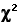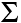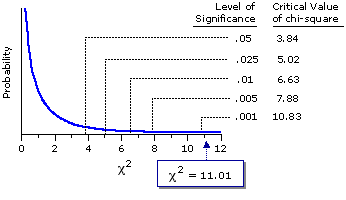Chapter 8.
Chi-Square Procedures for the Analysis of Categorical Frequency Data
Part 2

Chi-Square Procedures for Two Dimensions of Categorization

As noted at the beginning of this chapter, when observed items are sorted according to two or more separate dimensions of classification concurrently, they are said to be cross-categorized. The most efficient way to represent cross-categorized frequency data is with what is known as a contingency table. Suppose, for example, that there is a certain University—we will call it University Z, or UZ for short—which has several graduate professional schools. An investigator is interested in determining whether the students at two of these entities, the L-school and the B-school, differ in their respective proportions of liberals and conservatives. To this end, the investigator takes a random sample of students from each of the two schools and asks them which of the two labels they prefer as a self-description, "liberal" or "conservative." She then cross-categorizes the subjects according to (i) their school affiliation, L- or B-, and (ii) their preferred self-description as either "liberal" or "conservative." The resulting contingency table would have the following form, except that the various verbal descriptions beginning with the phrases "number of" and "total" would of course be replaced with specific numerical values.

 Preferred Self-Description Liberal Conservative School L-school number ofL-schoolliberals number ofL-schoolconservatives totalL-schoolsubjects B-school number ofB-schoolliberals number ofB-schoolconservatives totalB-schoolsubjects totalliberals totalconservatives totalsubjects

When chi-square procedures are applied to a contingency table of this sort, it is typically with the aim of determining whether the two categorical variables are associated; hence the name of this version, the chi-square test of association. If L-school and B-school subjects divided themselves as "liberal" and "conservative" in equal proportions, as in the following example

 Liberal Conservative L-school 55% ofL-school subjects 45% ofL-school subjects B-school 55% ofB-school subjects 45% ofB-school subjects

there would clearly be no evidence of association between the two variables. If on the other hand they divided themselves up in different proportions, for example

 Liberal Conservative L-school 55% ofL-school subjects 45% ofL-school subjects B-school 45% ofB-school subjects 55% ofB-school subjects

then there would be at least the suggestion of an association between the two variables. In this particular instance, the null hypothesis would be that there is no overall difference between L-school and B-school students at UZ with respect to how they divide themselves up on the liberal/conservative self-description. The chi-square test of association permits one to determine how likely it is that random samples of L-school and B-school students could have produced a liberal/conservative difference this large or larger, by mere chance coincidence, if the null hypothesis were true.

Here is a concrete example of cross-categorized frequency data, adapted from a medical study that sought to determine whether estrogen supplementation might delay or prevent the onset of Alzheimer's disease in postmenopausal women.
 M-X. Tang, D. Jacobs, Y. Stern, et al., "Effect of oestrogen during menopause on risk and age at onset of Alzheimer's disease." Lancet 1996; 348, 429-32.
The subjects in the study were 1,124 postmenopausal women who were initially free of Alzheimer's disease, Parkinson's disease, and stroke, and who were taking part in a longitudinal study of ageing and health in a New York City community. Of these women, 156 had received estrogen supplementation following the onset of menopause, while the remaining 968 had not. The following table breaks these two groups down according to whether the women did or did not show signs of Alzheimer's disease onset during a five-year follow-up period, as judged by annual clinical assessments and criterion-based diagnoses.

 Alzheimer's onsetduring 5-year period No Yes received estrogen Yes 147 9 156 No 810 158 968 957 167 1,124

In case the bare numbers in this table do not jump right out at you, here is the bottom line in percentage terms: Of the women who did not receive estrogen supplementation, 16.3% (158/968) showed signs of Alzheimer's disease onset during the five-year period; whereas, of the women who did receive estrogen supplementation, only 5.8% (9/156) showed signs of disease onset. It is not difficult to adduce reasons that might account for such a difference. As the authors of the study note, estrogen "promotes the growth of cholinergic neurons, stimulates the secretase metabolism of the amyloid precursor protein, and may interact with apolipoprotein E. All these factors could affect the risk of Alzheimer's disease." But of course, none of these possibilities amounts to anything at all unless we can establish that the difference between the observed percentages—16.3% versus 5.8%—reflects anything other than mere random variability. Once again it is the question of statistical significance: How likely is it that a difference this large or larger could have occurred by mere chance coincidence?

The procedures for applying chi-square to a two-dimensional situation of this sort are the same as we saw for the one-dimensional situation, except for two small modifications. The first has to do with what we take to be the MCE expected cell frequencies, and the second pertains to how we calculate the appropriate value for degrees of freedom. In both of these modifications the underlying logic is the same; it is only the details that differ.

In the one-dimensional chi-square test the values of E are simply stipulated in advance. In the fish example they were given as 100/100/100, because the sample was to be compared with the proportions of the three fish species that had been recorded over the preceding years. In the student survey example they were set to match the proportions of responses in the various categories that had been found in the large national survey. In the two-dimensional situation, on the other hand, the expected cell frequencies are not given in advance, nor are they intuitively obvious. We will illustrate the logic of the point with a simple if somewhat fanciful example.

Two friends, A and B, believe their friendship to be so deep as to produce a remarkable pattern of correspondences. Often they find that they are thinking the same thing at the same time. Often, even when separated, they find that they are doing the same thing at the same time. To put their faith to the test, they each toss a coin 100 times in succession, recording on each occasion the head/tail outcome of A's toss and the corresponding head/tail outcome of B's toss. Their hypothesis is that when A gets a head, B will also tend to get a head; and that when A gets a tail, B will also tend to get a tail. They of course do not suppose that even their relationship is so deep as to ensure these correspondences in 100 percent of the tosses, though they do believe that the pattern of such correspondences will significantly exceed what would be expected on the basis of mere chance coincidence.

For the sake of discussion, suppose that A and B each end up with exactly 50 heads and 50 tails. In that case the contingency table would have the following marginal totals, irrespective of how much or little the heads and tails outcomes of A and B might correspond.

 Outcomes for B Tails Heads Outcomes for A Heads [cell a] [cell b] 50 Tails [cell c] [cell d] 50 50 50 100

Actually, this is one of the rare scenarios for which the values of E would be fairly intuitively obvious. If you were asked to guess the values of the MCE expected frequencies for cells a, b, c, and d, I expect you would answer 25/25/25/25—and this would be quite correct. Now, if only you can make explicit the hidden logic that leads you to this answer, you will have the procedure for figuring out the values of E for two-dimensional chi-square situations in general. I suspect the core of your implicit logic runs something like this: If A gets 50 percent heads and B gets 50 percent tails; and if nothing other than mere chance coincidence is operating in the situation; then the (conjunctive) probability that any particular one of the 100 paired tosses will include a head for A and a tail for B is .5x.5=.25. Thus, the expected frequency for cell a is 25 percent of the total number of paired tosses: Ea=.25x100=25. The same logic would also lead you to Eb=25, Ec=25, and Ed=25.

Now see how the same logic can be extended to situations that are not intuitively obvious. When A and B perform their series of 100 paired tosses, it is actually not very likely that they would both end up with exactly 50 percent heads and 50 percent tails. It is much more likely that they each would end up with something slightly different from an exact 50/50 split. Suppose that A comes out with 46 heads and 54 tails, while B ends up with 48 heads and 52 tails. In this case the marginal totals would be distributed as follows

 Outcomes for B Tails Heads Outcomes for A Heads [cell a] [cell b] 46 Tails [cell c] [cell d] 54 52 48 100

and the logic would run like this. It is the same logic as outlined above, but now we will lay it out more formulaically. The proportion of paired tosses that include a head for person A is 46/100=.46; the proportion that include a tail for person B is 52/100. If nothing other than mere chance coincidence is operating in the situation, the probability that any particular one of the 100 paired tosses will include a head for A and a tail for B is therefore .46x.52=.2392; and the mean chance expected number of such conjunctions in 100 paired tosses is accordingly
 Ea = 46100 x 52100 x 100 = 23.92

This same structure can now be generalized to any situation at all where we have frequency data arranged in a rows by columns matrix. Defining "R" as the marginal total of the row to which the cell belongs, "C" as the marginal total of the column to which the cell belongs, and "N" as the total number of cross-categorized observations, we can write it as
 Ecell = R  N x C  N x N
which reduces algebraically to the simpler structure
 Ecell = RxCN That is: For each cell, multiply the marginal total for the row to which the cell belongs by the marginal total for the column to which the cell belongs, and then divide the result by the total number of cross-categorized observations.

The following illustration shows how this simple calculation works out for each of the cells of the present coin-toss example.

 Outcomes for B Tails Heads Outcomes for A Heads Ea = 46x52100= 23.92 Eb = 46x48100= 22.08 46 Tails Ec = 54x52100= 28.08 Ea = 54x48100= 25.92 54 52 48 100

And here is the same procedure applied to our estrogen/Alzheimer's example.

 Alzheimer's onsetduring 5-year period No Yes received estrogen Yes Ea = 156x9571124= 132.82 Eb = 156x1671124= 23.18 156 No Ec = 968x9571124= 824.18 Ed = 968x1671124= 143.82 968 957 167 1,124

Translation: The null hypothesis holds that estrogen supplementation in postmenopausal women makes no difference with respect to Alzheimer's onset. If this null hypothesis were true, then these are the frequencies we would have expected for the various cells of the table, given the marginal totals that appear along the right edge and the bottom of the table.

In case all this calculation and its accompanying jargon are proving a bit confusing, step back for a moment and think of it this way. On the null hypothesis, we would have expected the two categories of subjects—those who received estrogen supplementation and those who did not—to show approximately the same proportionate incidence of Alzheimer's onset during the five-year period. And that is exactly what the calculated values of E are specifying. Thus, of the 156 subjects who did receive estrogen supplementation, we would have expected a 23.18/156=14.9% rate of onset; and for the 968 subjects who did not receive supplementation, we would have expected an identical 143.82/968=14.9% rate. The same holds true for the expected rate of non-onset: 132.82/156=85.1% for those who received supplementation, and 824.18/968=85.1% for those who did not receive supplementation.

The next table shows these values of E, now listed in red, in comparison with the original values of O. Notice, incidentally, that the values of O and E, when summed across the rows and the columns, both add up to the same marginal totals.

 Alzheimer's onsetduring 5-year period No Yes received estrogen Yes 147 132.82 9 23.18 156 No 810 824.18 158 143.82 968 957 167 1,124

Beyond this point, the calculation of chi-square for the two-dimensional situation depends on the particular numbers of rows and columns. If there are more than two rows or more than two columns, it proceeds exactly as it does in the one-dimensional case, in accordance with the formula=(O—E)2E

When there are exactly two rows and two columns, the calculation requires a correction for continuity analogous to the correction described in Chapter 6 for binomial probability situations:=(|O — E|—.5)2E I.e., for each cell, subtract one-half unit from the absolute (unsigned) difference between O and E before squaring that difference.

As our current example has exactly two rows and two columns, the appropriate procedure is the latter. The following table shows the specific calculations for each of the four cells.

 Alzheimer's onsetduring 5-year period No Yes receivedestrogen Yes (|147—132.82|-.5)2132.82= 1.41 (|9—23.18|-.5)223.18= 8.07 No (|810—824.18|-.5)2824.18= 0.23 (|158—143.82|-.5)2143.82= 1.3 sum:= 11.01

The next step is to refer our calculated value of chi-square to the appropriate sampling distribution, which you will recall is defined by the applicable number of degrees of freedom. When applying chi-square procedures to situations in which there is only one dimension of categorization, the general principle for determining degrees of freedom is

df = (number of cells)1

With two dimensions of classification, it is a different formula but the same basic logic. Suppose you have two rows and two columns of cells, as shown below, and you are free to plug any integer numbers that you want into them, subject only to the stipulation that the cell values summed across rows, down columns, and overall, must add up to the fixed row, column, and overall sums that appear along the right edge and across the bottom.

 cell a = ? cell b = ? 50 cell c = ? cell d = ? 40 48 42 90

Here again your "freedom" is limited by the fixed sums. Arbitrarily plug 10 into cell a and the remaining three cells are instantly fixed at b=40, c=38, and d=2. Plugging 20 into cell d fixes the other cells at b=22, c=20, and a=28; and so on. For chi-square situations involving two rows and two columns of cells, degrees of freedom will in every instance be equal to one.

If you have two rows and three columns, your "freedom" is increased a bit, though still limited by the fixed sums.

 cell a = ? cell b = ? cell c = ? 50 cell d = ? cell e = ? cell f = ? 40 25 40 25 90

If you plug 10 arbitrarily into cell a, cell d becomes fixed at 15, but the other four cells remain "free" to vary. Plug some other number into any of these remaining four cells, however, and everything else becomes instantly fixed. For chi-square situations involving two rows and three columns (or three rows and two columns), degrees of freedom is in every case equal to two. More generally, when applying chi-square procedures to situations in which there are two dimensions of categorization, the general principle for determining degrees of freedom is
 df = (r—1)(c—1) r = number of rows c = number of columns

For our estrogen/Alzheimer's example, there are 2 rows and 2 columns. Hence

 df = (2—1)(2—1) = 1

Figure 8.6 shows the sampling distribution of chi-square for df=1, along with the critical values of chi-square for several levels of significance. As indicated, a chi-square value equal to or greater than 3.84 would be significant at or beyond the .05 level; a chi-square value equal to or greater than 5.02 would be significant at or beyond the .025 level; and so on.

Figure 8.6. Sampling Distribution of Chi-Square for df=1Our calculated value of=11.01 exceeds the value of chi-square (10.83) required for significance at the .001 level; hence we can say that the observed result is significant beyond the .001 level. That is: If the null hypothesis were true—if estrogen supplementation were unrelated to the onset of Alzheimer's disease in postmenopausal women—the likelihood of obtaining a differential onset rate as great as the one observed (16.3% versus 5.8%), by mere chance coincidence, would be smaller than one-tenth of one percent. Our investigators can therefore reject the null hypothesis with a high degree of confidence.

End of Chapter 8, Part 2.